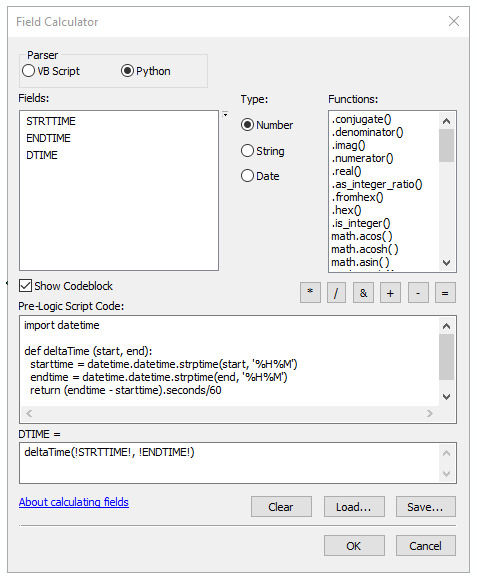# Calculate time duration from military time with ArcMap Field Calculator

396
1
07-15-2019 09:20 AMOccasional Contributor

I have two text fields (STRTTIME, ENDTIME) which are are formatted as military time with leading zeros. For example, 0540, 1655.

I want to calculate a new field with the time duration as integers (in minutes). How can I go about this using the Field Calculator in ArcMap?

1 Solution

Accepted SolutionsbyMVP Regular Contributor

You can use a Python function in field calculator to determine difference in time.  Since your data is in military time with leading zeros it is even easier.  Create a function as noted below.  This function takes the string parameters, convers them to datatime variables, calculates the difference in seconds and return this value multiplied by 60 to give you minutes. This script does assume the times are within a single 24-hour day and that STRTTIME is before ENDTIME.  It can be modified to include times on multiple days if the date is provided as well.

``````import datetime

def deltaTime (start, end):
starttime = datetime.datetime.strptime(start, '%H%M')
endtime = datetime.datetime.strptime(end, '%H%M')
return (endtime - starttime).seconds()/60‍‍‍‍‍‍‍‍‍‍‍‍‍‍‍‍‍‍‍‍‍‍‍‍‍‍‍‍‍‍‍‍‍‍‍‍‍‍‍‍‍‍‍‍‍‍‍``````

You can call this function in field calculator using deltaTime(!STRTTIME!, !ENDTIME!)byMVP Regular Contributor

You can use a Python function in field calculator to determine difference in time.  Since your data is in military time with leading zeros it is even easier.  Create a function as noted below.  This function takes the string parameters, convers them to datatime variables, calculates the difference in seconds and return this value multiplied by 60 to give you minutes. This script does assume the times are within a single 24-hour day and that STRTTIME is before ENDTIME.  It can be modified to include times on multiple days if the date is provided as well.

``````import datetime

def deltaTime (start, end):
starttime = datetime.datetime.strptime(start, '%H%M')
endtime = datetime.datetime.strptime(end, '%H%M')
return (endtime - starttime).seconds()/60‍‍‍‍‍‍‍‍‍‍‍‍‍‍‍‍‍‍‍‍‍‍‍‍‍‍‍‍‍‍‍‍‍‍‍‍‍‍‍‍‍‍‍‍‍‍‍``````

You can call this function in field calculator using deltaTime(!STRTTIME!, !ENDTIME!)